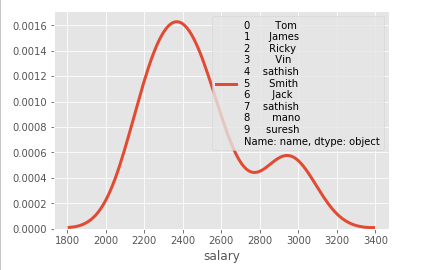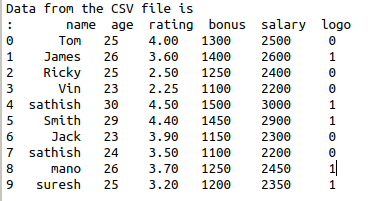• #5, First Floor, 4th Street Dr. Subbarayan Nagar Kodambakkam, Chennai-600 024 Landmark : Samiyar Madam
• pro@slogix.in
• +91- 81240 01111

### How to create density plot using seaborn library in python?

###### Description

To create density plot for X variable using python.

###### Process

Import seaborn library.

Put the density plot.

###### Sample Code

#import library functions

import seaborn as sns

import pandas as pd

Sathish/Pythonfiles/Employee.csv’)

#create data frame

df=pd.DataFrame(data)

print(“Data from the CSV file is\n”,df)

#density plot

sns.distplot(df[‘salary’], hist = False, kde = True,

kde_kws = {‘linewidth’: 3},label = df[‘name’])

###### Screenshots